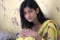## How to create transparent Status Bar and Navigation Bar in iOS?Sharon Christine

Updated on 30-Jul-2019 22:30:26

990 Views

You might have come across many application where the screen extends to complete screen i.e transparent Status Bar and transparent navigation bar.Here we will be seeing how to create an application where the you’ll be having transparent status and navigation bar.So let’s get startedStep 1 − Open Xcode → New ... Read MoreSharon Christine

Updated on 30-Jul-2019 22:30:26

466 Views

If you’re developing a game or a kids application or an application where you want to make attractive user interface you must know how to add shadow on text. This will not only makes the text attractive but also it will also enhance the user experience.Here we will see how ... Read More

## HTML DOM Input Month stepDown() MethodSharon Christine

Updated on 30-Jul-2019 22:30:26

28 Views

The HTML DOM input month stepDown() method steps down (decrement) the value of input month field by a specified value.SyntaxFollowing is the syntax −object.stepDown(number)Here, if number parameter is omitted then it decrements the value by 1.ExampleLet us see an example of input month stepDown() method − Live Demo HTML ... Read More

## HTML DOM Input Month stepUp() MethodSharon Christine

Updated on 30-Jul-2019 22:30:26

30 Views

The HTML DOM input month stepUp() method steps up (increment) the value of input month field by a specified value.SyntaxFollowing is the syntax −object.stepUp(number)Here, if number parameter is omitted then it increments the value by 1.ExampleLet us see an example of input month stepUp() method − Live Demo HTML ... Read More

## HTML DOM Input Month form PropertySharon Christine

Updated on 30-Jul-2019 22:30:26

45 Views

The HTML DOM input month form property returns the reference of the form that contains the month input field in the HTML document.SyntaxFollowing is the syntax −object.formExampleLet us see an example of HTML DOM input month form property − Live Demo    body{       text-align:center; ... Read More

## HTML DOM Input Number form PropertySharon Christine

Updated on 30-Jul-2019 22:30:26

45 Views

The HTML DOM input number form property returns the reference of the form that contains the number input field in the HTML document.SyntaxFollowing is the syntax −object.formExampleLet us see an example of HTML DOM input number form property − Live Demo body{ ... Read More

## Program to calculate area and perimeter of equilateral triangleSharon Christine

Updated on 30-Jul-2019 22:30:26

187 Views

Tringle is a closed figure with three sides. An equilateral triangle has all sides equal. Area and perimeter of an equilateral triangle can be found using the below formula, Area of equilateral triangle = (√3)/4*a2Perimeter of equilateral triangle = 3 * aLogic of CodeTo find the area of an equilateral ... Read More

## Program to calculate area and volume of a TetrahedronSharon Christine

Updated on 30-Jul-2019 22:30:26

170 Views

A tetrahedron is a pyramid with triangular base i.e. it has a base that is a triangle and each side has a triangle. All the three triangles converge to a point. As in the figure, Code Logic − The code to find the area and volume of tetrahedron uses the ... Read More

## Program to calculate area of EnneagonSharon Christine

Updated on 30-Jul-2019 22:30:26

70 Views

An enneagon also known as nonagon is a polygon with 9 sides. To calculate the area of enneagon the following formula is used, Area of ennagon = ((a2*9) / 4*tan (20°)) ∼= (6.18 * a2)Code Logic, The area of a polygon with nine sides is calculated by using the formula, ... Read More

## Program to calculate Area Of OctagonSharon Christine

Updated on 30-Jul-2019 22:30:26

238 Views

An octagon is a polygon with eight sides. To calculate the area of octagon the following formula is used, Area of octagon = ((a2*2) / *tan (22.5°)) = ((2*a*a)(1+√2))Code Logic, The area of a polygon with eight side is calculated by using the above formula. The expression uses sqrt function ... Read More